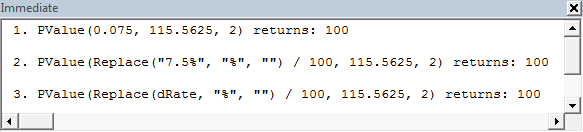# xlf Custom functions

## Function arguments with percentage and decimal format

Excel formulas accept percentage values in both decimal form and the percent operator. For example, 0.055, and 5.5% both return 0.055 when entered in a cell in Excel.

Consider the following formula for calculating the present value $PV$ from the future value $FV$ on an amount, at a discount rate $r$ over $n$ periods.

$$PV=FV(1+r)^{-n}$$

In code 1. the custom function for this formaula is named PValue.

The syntax is: PValue(DiscRate, FValue, NoPeriods), where DiscRate is the interest rate per period, FValue is the future value, and NoPeriods is the number of periods.

Code 1: A UDF that returns Present Value. DiscRate is in decimal form
Function PValue(DiscRate As Double, FValue As Double, NoPeriods As Double) As Double
PValue = FValue * (1 + DiscRate) ^ -NoPeriods
End Function


## Calling the PValue custom function from Excel

Using PValue in the Excel formula bar is straight forward, because Excel automatically accepts percentage values in decimal form and with with the percent operator.

The same applies to a custom function entered via the Function Wizard on the Formulas tab. In this example, the discount rate DiscRate is entered as 7.5%, and is stored in computer memory as 0.075 as shown in figure 1. DiscRate 7.5% = 0.075.

## Calling the PValue custom function from VBA

VBA does not automatically convert percentage values such as 7.5% to decimal format. In VBA the % character is a type declaration character for an integer.

The Sub procedure PValue_test in code 2 is used to illustrate the differences under three different scenarios.

• Scenario 1, the rate is in decimal format 0.075
• Scenario 2, the rate is in percentage format 7.5%, and
• Scenario 3, the rate of 7.5% is passed with a variable of the type String.

In each case, the output is sent to the Immediate Window with the Debug.Print statement.

Details of VBA functions:

• Replace: Returns a string in which a specified substring has been replaced with another substring a specified number of times.

• Val: Returns the numbers contained in a string as a numeric value of appropriate type.

Code 2: A procedure 1. decimal 0.075 - line 5, 2. 7.5% as String argument - line 8, and 3. 7.5% as String variable - line 13
Sub PValue_test()
Dim ans As Double
Dim dRate As String
'' Scenario 1
ans = PValue(0.075, 115.5625, 2)
Debug.Print "1. PValue(0.075, 115.5625, 2) returns: " & ans & vbNewLine
'' Scenario 2
ans = PValue(Replace("7.5%", "%", "") / 100, 115.5625, 2)
Debug.Print "2. PValue(Replace(""7.5%"", ""%"", """") / 100, 115.5625, 2) " & _
"returns: " & ans & vbNewLine
'' Scenario 3
dRate = "7.5%"
ans = PValue(Replace(dRate, "%", "") / 100, 115.5625, 2)
Debug.Print "3. PValue(Replace(dRate, ""%"", """") / 100, 115.5625, 2) " & _
"returns: " & ans & vbNewLine
End Sub


In scenario 1, line 5, the 0.075 is simply passed as a double to PValue and the return value is received by the calling procedure. In Scenario 2, line 8, and scenario 3, line 13, however, the 7.5% must be entered as a String and is enclosed with the "" expression.

Normally, the Val function would be used to extract the leading number sequence from the string, however, it does not work in this situation. The problem is that the % character is treated as the VBA Type Declaration Character for an Integer. This means that if "7.5%" is passed to the Val function, it only returns the integer component 7. It also returns a Type mismatch error. To prevent this, the Replace function is used to strip out (replace) the % character with nothing "". So Replace("7.5%") / 100 returns 0.075.

Another feature of code 2 is the inclusion of double quotes in a text string. In code 2, line 13, the "%" character is entered with double double quotes ""%"".

The Immediate Window with details for each scenario is shown in figure 2.Fig 2: The return values of the Debug.Print statement for each scenario
• This example was developed in Excel 2010 32 bit